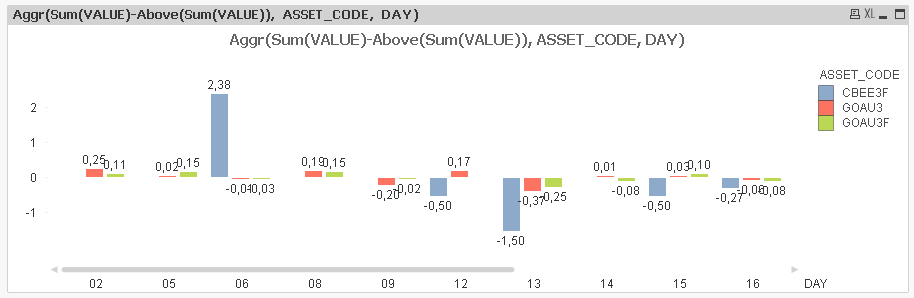# New to QlikView

Discussion board where members can get started with QlikView.

Contributor

## Chart with variation between days

Hi!

I have a chart with values per day and asset code. And I'd like to have the variation from one day to the other for each asset code.

how can I do it?

Tags (1)
1 Solution

Accepted SolutionsMVP

## Re: Chart with variation between days

Then try this:

Aggr(Sum({\$<[ANO DO PREGAO] = {'2016'},[MES PREGAO]={'09'}>}(VALUE)/100)-Above(Sum({\$<[ANO DO PREGAO] = {'2016'},[MES PREGAO]={'09'}>}(VALUE)/100)), ASSET_CODE, DAY)

4 RepliesMVP

## Re: Chart with variation between days

Is this the goal?Expression:

Aggr(Sum(VALUE)-Above(Sum(VALUE)), ASSET_CODE, DAY)

Contributor

## Re: Chart with variation between days

My origninal expression has some setanalysis, how can do as you did above in this case?

(SUM({\$<[ANO DO PREGAO] = {'2016'},[MES PREGAO]={'09'}>}(VALUE)/100))MVP

## Re: Chart with variation between days

Then try this:

Aggr(Sum({\$<[ANO DO PREGAO] = {'2016'},[MES PREGAO]={'09'}>}(VALUE)/100)-Above(Sum({\$<[ANO DO PREGAO] = {'2016'},[MES PREGAO]={'09'}>}(VALUE)/100)), ASSET_CODE, DAY)

Contributor

worked!

thanks